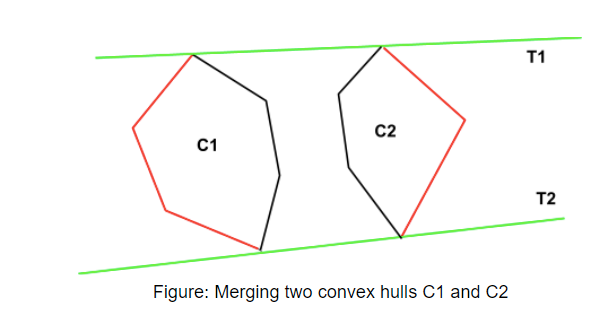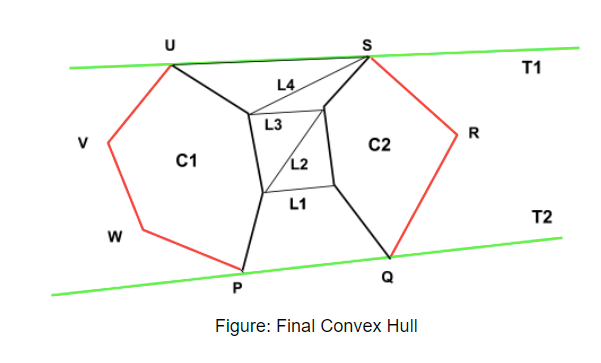# Solving The Convex Hull Problem using Divide and Conquer Algorithm

## Introduction

Convex Hull is a convex polygon having the smallest area and containing all points in the 2-D plane.

The problem states that we are given a set of points in a 2-D plane, and we have to find the convex hull of those points (i.e., return the vertices of the convex hull). We’ll try to come up with a divide and conquer solution for this problem.

I strongly recommend you to check out this blog if you want to know more about the working of Divide and Conquer algorithms. Moreover, the idea of this problem is very similar to the Merge Sort algorithm, which I have discussed in detail in that blog.

For an overview, the Divide and Conquer algorithm (or DAC) solves a very big task or a problem by breaking it into smaller sub-tasks or sub-problems.

## Algorithm

The steps that we’ll follow to solve the problem are:

1. First, we’ll sort the vector containing points in ascending order (according to their x-coordinates).
2. Next, we’ll divide the points into two halves S1 and S2. The set of points S1 contains the points to the left of the median, whereas the set S2 contains all the points that are right to the median.
3. We’ll find the convex hulls for the set S1 and S2 individually. Assuming the convex hull for S1 is C1, and for S2, it is C2.
4. Now, we’ll merge C1 and C2 such that we get the overall convex hull C.

Pretty straightforward, right!!

Here the only tricky part is merging the two convex hulls C1 and C2.

### Let’s see how we merge the sub-results.

The idea here is that the two tangents of the left and the right convex hulls (C1 and C2) will make the final convex hull C.The base case in the recursion will be when the number of points is less than or equal to 5 (i.e., n<=5); we will use the Brute-Force approach to find the solution. (Remember in merge sort, our base case was when the size of the array is one, we consider it as sorted)

## Finding the upper tangent and lower tangent.

Let L1 be the line that joins the rightmost point of the left convex hull C1 and the leftmost point of the right convex hull C2.

As L1 passes through the polygon C2, we take the anti-clockwise next point on C2 and label the line L2. The line is above the polygon C2, but it crosses the polygon C1, so we move to the clockwise next point, making the line L3. This again crosses the left polygon, so we move to line L4. This line is crossing the right polygon, so we move to line L5. Now, this final line is crossing neither of the points. Hence we get the upper tangent for the given two convex hulls.

For finding the lower tangent, we need to move inversely through the hulls, i.e., if the line is crossing the convex hull C2, we move to clockwise next and to anti-clockwise next if the line is crossing the convex hull C1Here, PQRSUVW is the convex hull of the set of given points.

## Implementation

``````#include<bits/stdc++.h>
using namespace std;
pair<int, int> mid;

// Function to find the quadrant where the point p lies
{
if (p.first >= 0 && p.second >= 0){
return 1;
}
if (p.first <= 0 && p.second >= 0){
return 2;
}
if (p.first <= 0 && p.second <= 0){
return 3;
}
else{
return 4;
}
}

// Function to check whether the line is crossing the polygon or not
int orient(pair<int, int> a, pair<int, int> b, pair<int, int> c)
{
int r = (b.second-a.second)*(c.first-b.first) - (c.second-b.second)*(b.first-a.first);
if (r == 0){
return 0;
}
if (r > 0){
return 1;
}
else{
return -1;
}
}

// Sorting of the points in Brute Force solution is dont using this function
bool mycompare(pair<int, int> point1, pair<int, int> point2)
{
pair<int, int> p = make_pair(point1.first - mid.first, point1.second - mid.second);
pair<int, int> q = make_pair(point2.first - mid.first, point2.second - mid.second);

if (o != t){
return (o < t);
}

return (p.second*q.first < q.second*p.first);
}

// Finds upper tangent of two polygons 'c1' and 'c2' represented as two vectors.
vector<pair<int, int>> merger(vector<pair<int, int> > c1, vector<pair<int, int> > c2)
{

int n1 = c1.size(), n2 = c2.size();
int ia = 0, ib = 0;
for (int i=1; i<n1; i++){
if (c1[i].first > c1[ia].first){
ia = i;
}
}

// ib -> leftmost point of b
for (int i=1; i<n2; i++){
if (c2[i].first < c2[ib].first){
ib=i;
}
}
// finding the upper tangent
int inda = ia, indb = ib;
bool done = 0;
while (!done)
{
done = 1;
while (orient(c2[indb], c1[inda], c1[(inda+1)%n1]) >=0)
inda = (inda + 1) % n1;
while (orient(c1[inda], c2[indb], c2[(n2+indb-1)%n2]) <=0)
{
indb = (n2+indb-1)%n2;
done = 0;
}
}
int uppera = inda, upperb = indb;

//finding the lower tangent
inda = ia, indb=ib;
done = 0;
int g = 0;
while (!done)
{
done = 1;
while (orient(c1[inda], c2[indb], c2[(indb+1)%n2])>=0)
indb=(indb+1)%n2;
while (orient(c2[indb], c1[inda], c1[(n1+inda-1)%n1])<=0)
{
inda=(n1+inda-1)%n1;
done=0;
}
}
int lowera = inda, lowerb = indb;
vector<pair<int, int>> ret;
int ind = uppera;
ret.push_back(c1[uppera]);
while (ind != lowera)
{
ind = (ind+1)%n1;
ret.push_back(c1[ind]);
}
ind = lowerb;
ret.push_back(c2[lowerb]);
while (ind != upperb)
{
ind = (ind+1)%n2;
ret.push_back(c2[ind]);
}
return ret;
}

// Brute force algorithm to find convex hull for a set of less than 6 points
vector<pair<int, int>> bruteHull(vector<pair<int, int>> c)
{
set<pair<int, int> >s;
for (int i=0; i<c.size(); i++)
{
for (int j=i+1; j<c.size(); j++)
{
int x1 = c[i].first, x2 = c[j].first;
int y1 = c[i].second, y2 = c[j].second;
int a1 = y1-y2;
int b1 = x2-x1;
int c1 = x1*y2-y1*x2;
int pos = 0, neg = 0;
for (int k=0; k<c.size(); k++)
{
if (a1*c[k].first+b1*c[k].second+c1 <= 0){
neg++;
}
if (a1*c[k].first+b1*c[k].second+c1 >= 0){
pos++;
}
}
if (pos == c.size() || neg == c.size())
{
s.insert(c[i]);
s.insert(c[j]);
}
}
}
vector<pair<int, int>>ret;
for (auto e:s){
ret.push_back(e);
}

mid = {0, 0};
int n = ret.size();
for (int i=0; i<n; i++)
{
mid.first += ret[i].first;
mid.second += ret[i].second;
ret[i].first *= n;
ret[i].second *= n;
}
sort(ret.begin(), ret.end(), mycompare);
for (int i=0; i<n; i++){
ret[i] = make_pair(ret[i].first/n, ret[i].second/n);
}

return ret;
}

// Function to find the convex hull for a set of points
vector<pair<int, int>> divide(vector<pair<int, int>> a)
{

if (a.size() <= 5){
return bruteHull(a);
}

vector<pair<int, int>> left, right;

for (int i=0; i<a.size()/2; i++){
left.push_back(a[i]);
}
for (int i=a.size()/2; i<a.size(); i++){
right.push_back(a[i]);
}

// Finding convex hull for the left and right sets
vector<pair<int, int>> left_convex_hull = divide(left);
vector<pair<int, int>> right_convex_hull = divide(right);

// Merging the convex hulls to get the final result
return merger(left_convex_hull, right_convex_hull);
}

int main()
{
vector<pair<int, int> > a;
int n;
cin>>n;
for(int i=0; i<n; i++){
int x, y;
cin>>x;
cin>>y;
a.push_back(make_pair(x, y));
}

// sorting the set of points according to the x-coordinate
sort(a.begin(), a.end());
vector<pair<int, int>> ans = divide(a);
cout << "The points in the convex hull are:\n";
for (auto e:ans)
cout << e.first << " "
<< e.second << endl;
return 0;
}``````

Sample Input

``````10
0 0
1 -4
-1 -5
-5 -3
-3 -1
-1 -3
-2 -2
-1 -1
-2 -1
-1 1``````

Sample Output

``````The points in the convex hull are:
-5 -3
-1 -5
1 -4
0 0
-1 1``````

## Complexity Analysis

### Time Complexity

The best-case, worst-case, and average-case time complexity of this approach is O(n*logn) because the merging of the two convex hulls takes O(n) time, and we are diving the set of points into left and right halves, so it takes O(logn) time, hence in total, the algorithm will take O(n*logn) time.

### Space Complexity

The space complexity of this divide and conquer approach for solving the convex hull problem will be O(n) because we are utilizing extra space.

1). What are the applications of the convex hull problem?

Answer: The problem has various applications, which include:

• Geographic Information Systems
• Image Processing
• Pattern Recognition
• Game Theory

2). What is the time complexity to solve the convex hull problem?

Answer: It takes O(n3) time using the brute force approach, whereas the divide and conquer approach takes O(n) time to find the convex hull. The divide and conquer algorithm is preferred here because it solves the problem in linear time and saves a lot of computation cost.

3). What are the Divide and Conquer algorithm?

Answer: The Divide and Conquer algorithm (or DAC) solves a very big task or a problem by breaking it into smaller sub-tasks or sub-problems; after solving, we combine all the sub-tasks in a specific manner so that we get the result for the big task.

4). List the advantages of the Divide and Conquer approach for the Convex Hull Problem.

• The Divide and Conquer method solves the problem in O(n*logn) time, whereas the Brute-Force solution takes up O(n3) time to solve the Convex Hull problem.
• The DAC algorithm is ideal for multi-processing systems because it inhibits parallelism. It is also fast because it makes excellent use of cache memory without shifting too much computation to main memory, which is relatively slower.
• The Divide and conquer algorithm divides the problem into smaller sub-problems; hence it is recommended for more complex problems like finding a convex hull for a set of points. The sub-problems can be executed on different processors making it more time-efficient.

## Key Takeaways

If you are worried about the upcoming Campus Placements, then don’t worry. Coding Ninjas has got you covered. A precisely crafted and designed course on interview preparation awaits you; just click on this link.

With this course, you can:

• Find out where you stand and get recommendations on moving towards your dream job.
• Understand your strengths & weaknesses with our 360-degree evaluation.
• Prepare yourself for non-tech topics and soft skills.

Happy Learning!